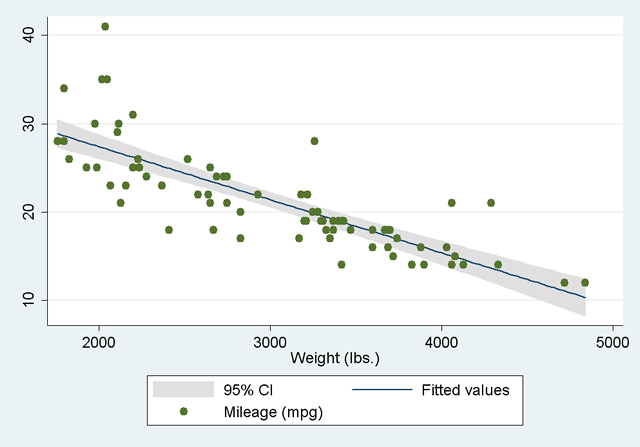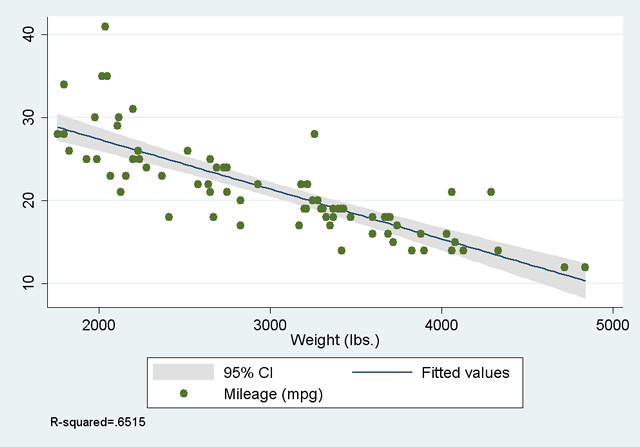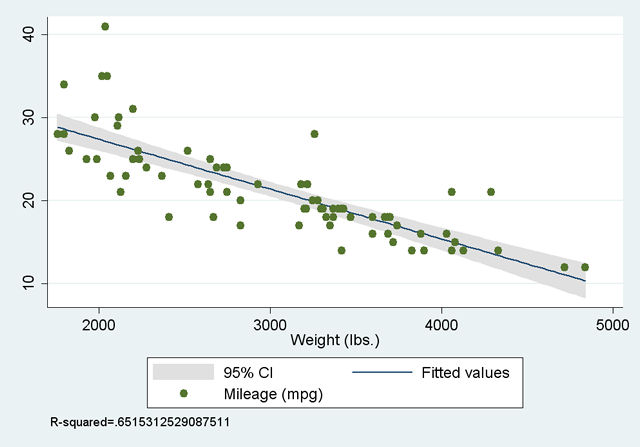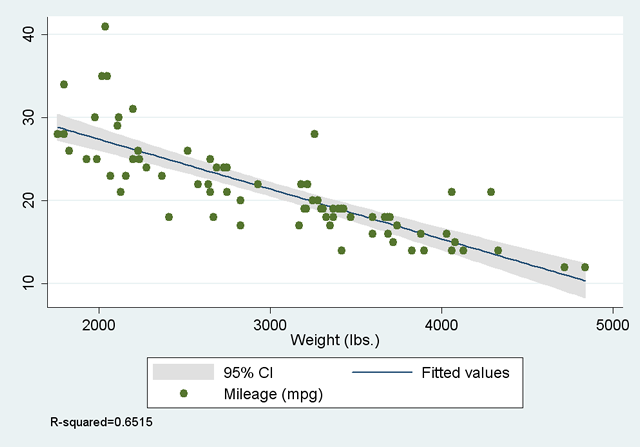# Including Calculated Results In Stata Graphs

Sometimes you want to include a result you've calculated in a Stata graph. For example, you might want to have a note give the mean of a variable. One option of course is to find out the mean ahead of time and just type it in. But what if your data change? Like all Stata commands, graph commands can include macros. So if you have Stata find the number you want and then store it as a macro, you can then use the macro to put that number in your graph.

If you're not familiar with macros, please read the Local Macros section of Stata Programming Essentials before continuing.

As an example, load the automobile data set. Then create a scatter plot of mpg vs. weight with a linear fit line overlaid on it, with confidence intervals:

sysuse auto
twoway (lfitci mpg weight) (scatter mpg weight)

Be sure to put the linear fit first so the points from the scatter plot can overlay the shaded 95% confidence interval.Next add a note giving the R-squared of the implied regression. Find it with:

regress mpg weight

which gives:

```      Source |       SS       df       MS              Number of obs =      74
-------------+------------------------------           F(  1,    72) =  134.62
Model |   1591.9902     1   1591.9902           Prob > F      =  0.0000
Residual |  851.469256    72  11.8259619           R-squared     =  0.6515
-------------+------------------------------           Adj R-squared =  0.6467
Total |  2443.45946    73  33.4720474           Root MSE      =  3.4389

------------------------------------------------------------------------------
mpg |      Coef.   Std. Err.      t    P>|t|     [95% Conf. Interval]
-------------+----------------------------------------------------------------
weight |  -.0060087   .0005179   -11.60   0.000    -.0070411   -.0049763
_cons |   39.44028   1.614003    24.44   0.000     36.22283    42.65774
------------------------------------------------------------------------------
```

You could just add a note with that value:

twoway (lfitci mpg weight) (scatter mpg weight), note(R-squared=.6515)But why not let Stata do the work? After a regression, the R-squared is stored as e(r2) . You can store that in a macro and put the macro in your graph instead of typing the number yourself.

(To see a full list of the stored values available to you, type ereturn list after an estimation command like this one, or return list after a basic command like summarize.)

local r2=e(r2)
twoway (lfitci mpg weight) (scatter mpg weight), note(R-squared=`r2')It works, but it's rather ugly because the r2 macro contains all sixteen digits. Four was much better. Fortunately there's a way to format a number before it's stored in a macro. It's done by calling on the display command:

local r2: display %5.4f e(r2)

Here we use a colon (local r2:) to indicate we're going to store the results of a display command in the local macro r2. %5.4f is the format we'll use: it specifies that the number be displayed with no more than five characters (including the decimal point) and no more than four digits to the right of the decimal point. As a result r2 contains .6515 rather than .6515312529087511, giving a much nicer result when you create the graph.

The complete code to create the nice graph is:

sysuse auto
reg mpg weight
local r2: display %5.4f e(r2)
twoway (lfitci mpg weight) (scatter mpg weight), note(R-squared=`r2')Last Revised: 5/25/2007